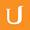## DF - Marginal Totals - Intro to Inferential Statistics

• 0:00 - 0:06
Let's pick any number in here. Let's say 5. Can we choose this number and still
• 0:06 - 0:13
have this sum to 9, and still have these columns sum to 9? Yeah. Let's say 3.
• 0:13 - 0:18
So together those make 8, and that means this one is forced it has to be 1.
• 0:18 - 0:24
Okay, can we pick a value here. Yeah, let's say 8. So those sum to 13, which
• 0:24 - 0:30
means that this value is forced. This has to be negative 4, and then can we
• 0:30 - 0:36
pick a value here, and have this row and this column still sum to 9? Yeah,
• 0:36 - 0:41
let's say 7. Now if this column has to sum to 9, then this entry's forced, it's
• 0:41 - 0:48
negative 1. And as you can see, this entry's forced too, this adds to 15, so to
• 0:48 - 0:55
add to 9, this should be negative 6. And this entry's forced as well, this has
• 0:55 - 1:01
to be 14. Then both this column and this row sum to 9. So in this case there
• 1:01 - 1:06
are 4 degrees of freedom. But if we have an n by n table, in this case this is
• 1:06 - 1:12
a 3 by 3 table. This is a 4 by 4 table. Then we would be able to chose all of
• 1:12 - 1:18
these entries but then these ones would be forced. This number of tiles is n
• 1:18 - 1:25
minus 1. And this number of tiles is also n minus 1. So the total number that
• 1:25 - 1:32
we can choose is n minus 1 squared. So here in this 3 by 3 table, we were able
• 1:32 - 1:39
to choose 2 times 2. In this 4 by 4 table, we were able to choose 3 times 3. So
• 1:39 - 1:44
when we have an n by n table, we can choose n minus 1 times n minus 1, or just
• 1:44 - 1:48
n minus 1 squared.
Title:
DF - Marginal Totals - Intro to Inferential Statistics
Description:

more » « less
Video Language:
English
Team:Udacity
Project:
UD201 - Intro to Inferential Statistics
Duration:
01:48Udacity Robot edited English subtitles for 06-11 DF - Marginal TotalsUdacity Robot edited English subtitles for 06-11 DF - Marginal TotalsUdacity Robot edited English subtitles for 06-11 DF - Marginal TotalsUdacity Robot edited English subtitles for 06-11 DF - Marginal TotalsCogi-Admin added a translation

# English subtitles

## Revisions Compare revisions

• API
Udacity Robot
• API
Udacity Robot
• API
Udacity Robot
• API
Udacity Robot